# How to create A dynamic named range expands automatically in Excel

Feb 01, 2020 • edited Feb 02, 2020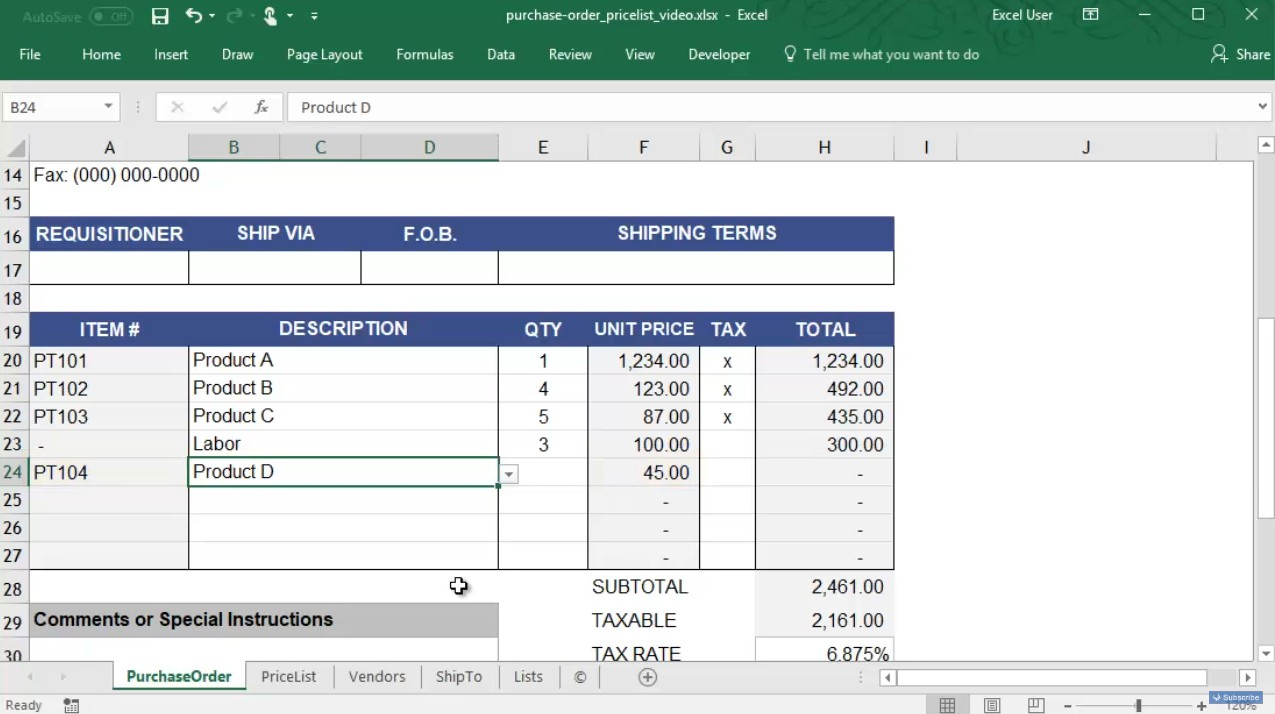In Microsoft Excel, you may have a named range that must be extended to include new information.

A dynamic named range expands automatically when you add a value to the range.

1. For example, select the range A1:A4 and name it Prices.

2. Calculate the sum.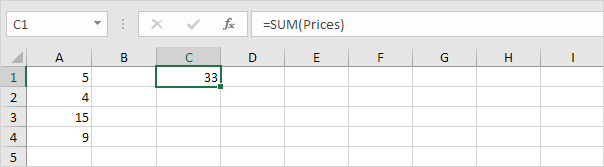3. When you add a value to the range, Excel does not update the sum.To expand the named range automatically when you add a value to the range, execute the following the following steps.

4. On the Formulas tab, in the Defined Names group, click Name Manager.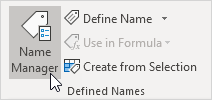5. Click Edit.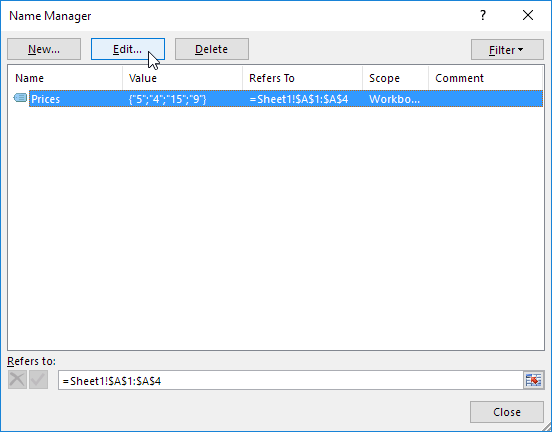6. Click in the “Refers to” box and enter the formula =OFFSET(\$A\$1,0,0,COUNTA(\$A:\$A),1)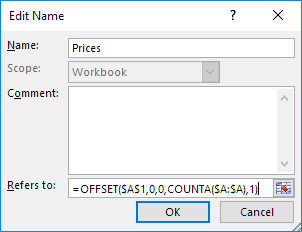Explanation: the OFFSET function takes 5 arguments. Reference: \$A\$1, rows to offset: 0, columns to offset: 0, height: COUNTA(\$A:\$A) and width: 1. COUNTA(\$A:\$A) counts the number of values in column A that are not empty. When you add a value to the range, COUNTA(\$A:\$A) increases. As a result, the range returned by the OFFSET function expands.

7. Click OK and Close.

8. Now, when you add a value to the range, Excel updates the sum automatically.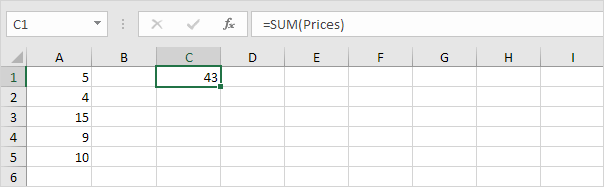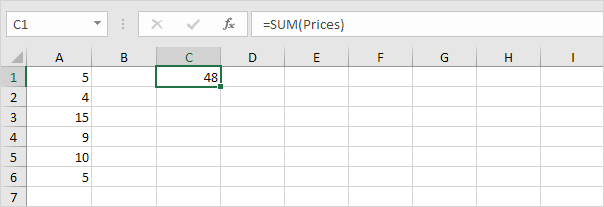#How To#Tutorial#Formulas#Functions

How To View And Customize The Status Bar In Excel

The various paste options in Excel